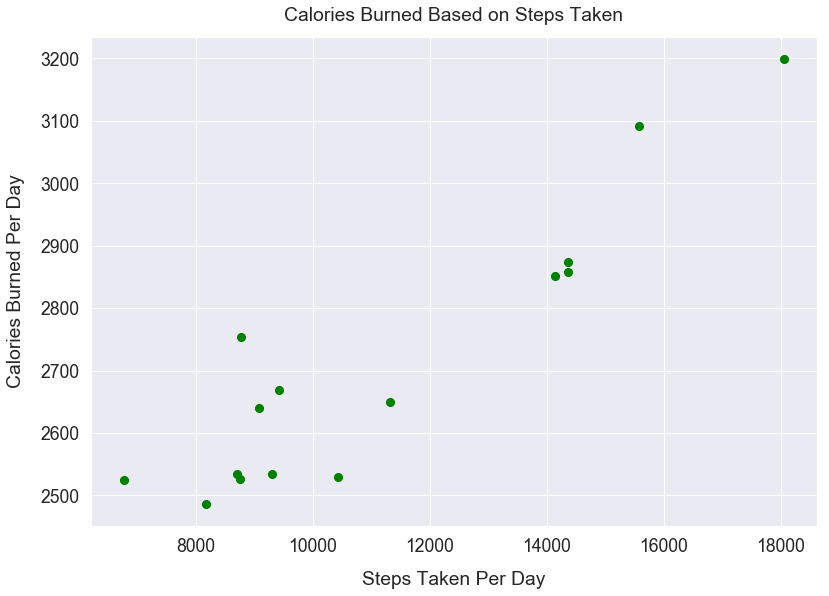Data Visualizations Matplotlib Plotting Tutorial

# Style Plots using Matplotlib

In this post, I'll walk through several small changes to style your plots in Matplotlib such as:

• Add X and Y axis labels
• Adjust individual font sizes of plot elements
• Adjust the color of scatter points
• Adjust all font sizes in a simple line
• Make scatter points larger

#### Import Modules¶

In :
import matplotlib.pyplot as plt
import seaborn as sns
% matplotlib inline


#### Fitbit Activity Data¶

Below is my Fitbit data of daily steps taken and daily calories burned over a 15-day period.

In :
calories_burned = [3092, 2754, 2852, 2527, 3199, 2640, 2874, 2649,
2525, 2858, 2530, 2535, 2487, 2534, 2668]

In :
steps = [15578, 8769, 14133, 8757, 18045, 9087, 14367, 11326, 6776,
14359, 10428, 9296, 8177, 8705, 9426]


#### Our Initial Plot with Default Styles¶

In :
plt.scatter(steps, calories_burned);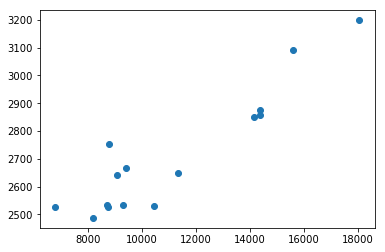There's a lot we can improve to make this plot more appealing.

Call the title method and pass in a string value for our title name.

In :
plt.scatter(steps, calories_burned)
plt.title("Calories Burned Based on Steps Taken");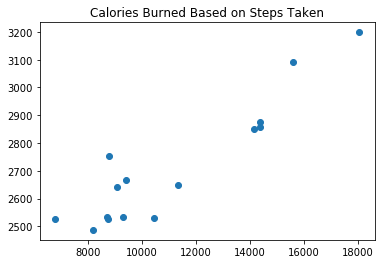#### Add X and Y Axis Labels¶

Call the xlabel method and pass in a string value for the label name on the x-axis.

Call the ylabel method and pass in a string value for the label name on the y-axis.

In :
plt.scatter(steps, calories_burned)
plt.title("Calories Burned Based on Steps Taken")
plt.xlabel("Steps Taken Per Day")
plt.ylabel("Calories Burned Per Day");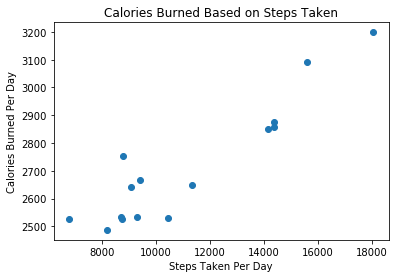Call the figure method and for the figsize argument, pass in a tuple of (width, height).

In :
plt.figure(figsize=(8, 8))
plt.scatter(steps, calories_burned)
plt.title("Calories Burned Based on Steps Taken")
plt.xlabel("Steps Taken Per Day")
plt.ylabel("Calories Burned Per Day");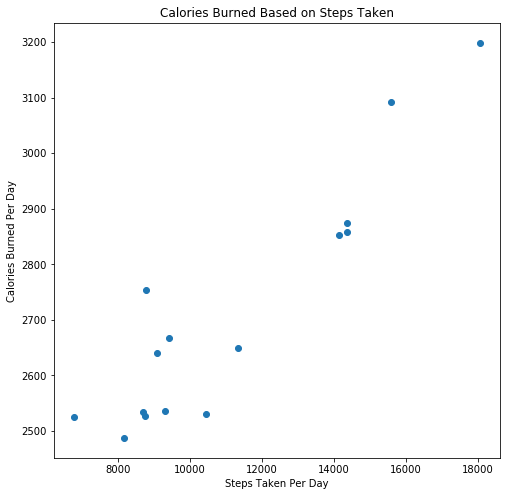For the methods title, xlabel, ylabel, include a numeric value for fontsize argument to change the font size.

Call the tick_params method and for the labelsize argument, pass in a numeric value to change the font size of the tick values.

Generally, tick values have smaller fonts than axes labels.

In :
plt.figure(figsize=(8, 8))
plt.scatter(steps, calories_burned)
plt.title("Calories Burned Based on Steps Taken", fontsize=18)
plt.xlabel("Steps Taken Per Day", fontsize=14)
plt.ylabel("Calories Burned Per Day", fontsize=14)
plt.tick_params(labelsize=12);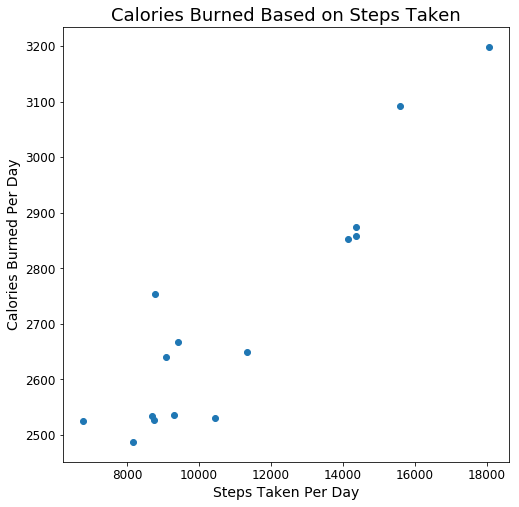Include the argument y in the title method and pass in a numeric value.

In :
plt.figure(figsize=(8, 8))
plt.scatter(steps, calories_burned)
plt.title("Calories Burned Based on Steps Taken", fontsize=18, y=1.02)
plt.xlabel("Steps Taken Per Day", fontsize=14)
plt.ylabel("Calories Burned Per Day", fontsize=14)
plt.tick_params(labelsize=12);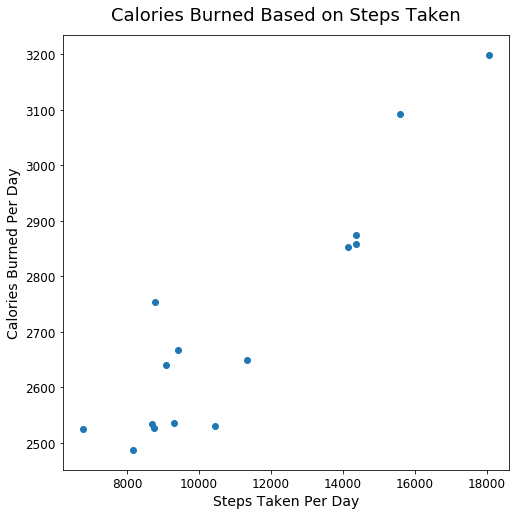In the tick_params method, for the argument pad, pass in a numeric value, being the pixel size betwen the tick values and the plot.

In :
plt.figure(figsize=(8, 8))
plt.scatter(steps, calories_burned)
plt.title("Calories Burned Based on Steps Taken", fontsize=18, y=1.02)
plt.xlabel("Steps Taken Per Day", fontsize=14)
plt.ylabel("Calories Burned Per Day", fontsize=14)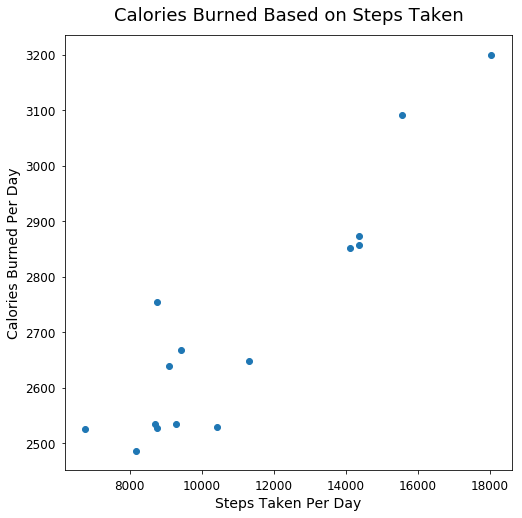In the xlabel and ylabel methods, include the argument labelpad and include a numeric value to add more padding.

In :
plt.figure(figsize=(8, 8))
plt.scatter(steps, calories_burned)
plt.title("Calories Burned Based on Steps Taken", fontsize=18, y=1.02)
plt.xlabel("Steps Taken Per Day", fontsize=14, labelpad=15)
plt.ylabel("Calories Burned Per Day", fontsize=14, labelpad=15)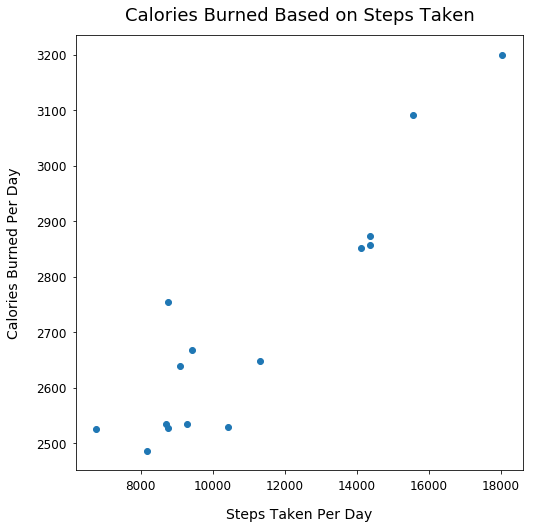#### Adjust the Color of our Scatter Points¶

In the scatter method, pass the value green to the c argument that specifies the color of our scatter points.

In :
plt.figure(figsize=(8, 8))
plt.scatter(steps, calories_burned, c='green')
plt.title("Calories Burned Based on Steps Taken", fontsize=18, y=1.02)
plt.xlabel("Steps Taken Per Day", fontsize=14, labelpad=15)
plt.ylabel("Calories Burned Per Day", fontsize=14, labelpad=15)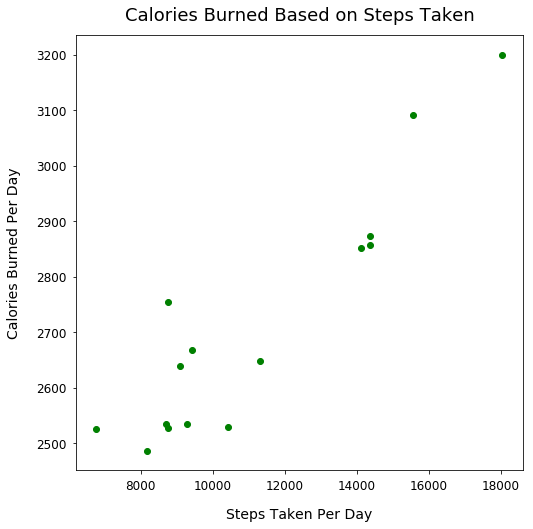#### Make Font Sizes and Plot Much Larger¶

Often times, the default size of plots and text in Matplotlib make it difficult to read. We can easily change all that with just 2 lines of code.

In the first line below, we declare sns.set(font_scale=1.6). Note, this uses the Seaborn visualization library, which is a wrapper on top of Matplotlib. These libraries work well together. This method call changes the background to be a light gray and adds a white grid. Also, this call scales all the fonts to be 1.6x greater than the default. I removed our previous individual font size calls for elements too.

Since most other elements are larger, I also made the figure size larger.

In :
sns.set(font_scale=1.6)
plt.figure(figsize=(13, 9))
plt.scatter(steps, calories_burned, c='green')
plt.title("Calories Burned Based on Steps Taken", y=1.02)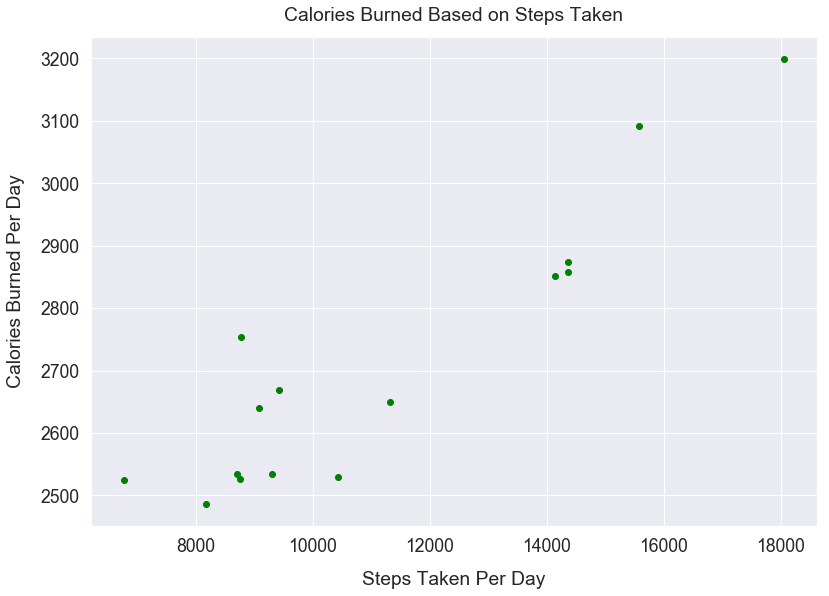#### Make Scatter Points Larger¶

Above, the size of the scatter points are very small. Let's make them larger so they're easier to spot on this large plot.

In the scatter method, pass the value 65 to the s argument that specifies the size of our scatter points.

In :
sns.set(font_scale=1.6)
plt.figure(figsize=(13, 9))
plt.scatter(steps, calories_burned, c='green', s=65)
plt.title("Calories Burned Based on Steps Taken", y=1.02)